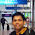Type Conversion - DotNet and DataBase## Tuesday, 3 July 2018

Type conversion is the process of converting one type of data into another type of data.
This is also called as Type casting.

This is two types

1) Implicit conversion
2) Explicit conversion

Implicit Conversion/Boxing
----------------------------------

Implicit conversion is the process of converting one datatype into another data type directly by assigning one type of  variable to another type of variable.

sbyte a = 10;
long b = a;
Console.WriteLine("Employee id is : " + b);

here sbyte and long are two different data types we are able to assign variable "a" to "b" directly.

The below listed datatypes we can implicitly convert

As per above table, we can convert sbyte to short,int,long,float,double and decimal
datatypes without using any conversion techniques.

Explicit conversion/Unboxing
----------------------------

Explicit conversion is also converting one type of variable into another type but here we suggest compiler to convert into another datatype.

This is two types

1) alias.parse
2) Convert class

1) alias.parse
---------------

Here alias is the datatype.
In the alias.parse method we ask compiler to convert string type of data into another type of data and alias is the datatype as it is not possible to convert by assigning directly.

string a = "10";
int b = int.Parse(a); // here int is the alias
Console.WriteLine("Employee id is : " + b);

alias.Parse is strict on type conversion.
In case the value supplied to "a" is not suitable to convert into int, it throws the run time error.

This we can overcome using "alias.TryParse" method as below.

string a = "10";
int b = 0;
int.TryParse(a, out b);
Console.WriteLine("Employee id is : " + b);

Here the value assigned to "a" is supports to convert into int, "b" value becomes 10 otherwise remains as 0.

string a = "10a";
int b = 0;
int.TryParse(a, out b);
Console.WriteLine("Employee id is : " + b);

Here it is not possible to convert "10a" into integer. So b value remains as 0.

2) Convert class
----------------

.Net framework provides some predefined methods for converting one datatype information into another datatype information.

Note: In case we convert any large datatype information into small datatype information, there is a chance for loss of data.

#### 1 comment:

1.Nice information Naga.

x